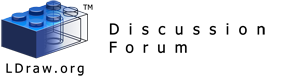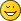Points on a circle primitive - Printable Version +- LDraw.org Discussion Forums (https://forums.ldraw.org) +-- Forum: LDraw Programs (https://forums.ldraw.org/forum-7.html) +--- Forum: Parts Author Tools (https://forums.ldraw.org/forum-24.html) +--- Thread: Points on a circle primitive (/thread-2974.html) Points on a circle primitive - Tim Gould - 2012-01-08 Hi all, Below is a perl script to take a bunch of angles and convert them to points on the edge of a circle primitve (ie. a 16 or 48 point polygon). The comments up top describe how to use it. It's designed to make generating rims on the bottom of curved parts relatively easy when there's only a few segments required and hand-coding makes more sense than using Philo's wonderful toys. Tim Code:```#! /usr/bin/perl # ang2point.pl [-r RAD] [-t THICK] [-s SEGS] ang1 ang2 ... angn # # RAD defaults to 20 # THICK defaults to none # SEGS defaults to 16 # # Projects the angles onto a circular primitive of radius RAD # with SEGS segments. If THICK is specified it also shows the # points on an inner radius of RAD-THICK # # It scales the radius at each angle to ensure it lies on the # SEG-sided polygon that approximates the circle. # use POSIX; \$rad=20; \$segs=16; @angs=(); \$thick=0; for (\$ia=0; \$ia<=\$#ARGV; \$ia++) {     \$opt=lc(\$ARGV[\$ia]);     if (\$opt eq '-r') {     \$ia++;     \$rad=\$ARGV[\$ia];     } elsif (\$opt eq '-t') {     \$ia++;     \$thick=\$ARGV[\$ia];     } elsif (\$opt eq '-s') {     \$ia++;     \$segs=\$ARGV[\$ia];     } else {     push @angs, \$opt;     } } \$nang=\$#angs+1; print "0 // ang2point.pl ".join(' ', @ARGV)."\n"; print "0 // Calculating \$nang points at outer radius \$rad on \$segs segments\n"; if (\$thick>0) {     \$radp=\$rad-\$thick;     print "0 // Also calculating inner radius \$radp\n"; } @reffs=(); @rieffs=(); \$kk=3.141592635/180; foreach \$ang (@angs) {     \$f=\$ang/360;     \$a0=floor(\$f*\$segs)/\$segs*360;     \$ar=\$ang-\$a0;     #print "\$a0 + \$ar\n";     \$c0=cos(\$kk*\$a0);     \$s0=sin(\$kk*\$a0);     \$cf=cos(\$kk*(\$a0+360/\$segs));     \$sf=sin(\$kk*(\$a0+360/\$segs));     \$ct=cos(\$kk*\$ang);     \$st=sin(\$kk*\$ang);     \$scale=(\$c0*\$sf-\$s0*\$cf)/((\$sf-\$s0)*\$ct-(\$cf-\$c0)*\$st);     \$reff=\$scale*\$rad;     \$rieff=\$scale*(\$rad-\$thick);     #print "Effective radius \$reff\n";     push @reffs, \$reff;     push @rieffs, \$rieff; } @pts=(); @ipts=(); foreach \$ia (0..\$#angs) {     \$x=\$reffs[\$ia]*cos(\$kk*\$angs[\$ia]);     \$z=\$reffs[\$ia]*sin(\$kk*\$angs[\$ia]);     push @pts, sprintf("0 // Point %2d: %.3f 0 %.3f\n",                \$ia+1, \$x, \$z);     if (\$thick>0) {     \$x=\$rieffs[\$ia]*cos(\$kk*\$angs[\$ia]);     \$z=\$rieffs[\$ia]*sin(\$kk*\$angs[\$ia]);     push @ipts, sprintf("0 // Inner point %2d: %.3f 0 %.3f\n",                 \$ia+1, \$x, \$z);     } } print @pts; print @ipts;``` Re: Points on a circle primitive - Philippe Hurbain - 2012-01-09 Looks interesting - I agree that my "wonderful" tools can be clumsy to useWould you have a sample run (not so familar with perl...) Re: Points on a circle primitive - Tim Gould - 2012-01-09 Not so much clumsy in this case as not quite the right tool for the job. That said I'll be using ytruder for certainI plan to demonstrate it properly when I get a chance to redo the base of 65188. I'll try to remember to snap the stages. But... Code:```0 // ang2point.pl -r 120 -t 4 -s 48 0 7.5 11 0 // Calculating 3 points at outer radius 120 on 48 segments 0 // Also calculating inner radius 116 0 // Point  1: 120.000 0 0.000 0 // Point  2: 118.973 0 15.663 0 // Point  3: 117.544 0 22.848 0 // Inner point  1: 116.000 0 0.000 0 // Inner point  2: 115.008 0 15.141 0 // Inner point  3: 113.626 0 22.087``` Tim PS. This one should be very easy to convert to C. But processing arguments is simpler in perl so I chose that option. Re: Points on a circle primitive - Tim Gould - 2012-01-09 And to follow up: Attached is the stages I took. Finally I ran ytruder on it